# Monday August 28th

## More Mathematica and pains with Excel

• So…I really need to learn some Excel. That’s all I’ll say on that. I plan to do so at the end of November, after my Nanodegree ends. I need to get better and making functions and filtering data in Excel. I have a self-paced course in mind from EdX. I never really learned that stuff. It’s important, expecially for understanding and analyzing data.

## I start Advanced C++ in two days!

• Yeah…so that’s going to be fun…my Desktop is crapping out on me, but I have another Desktop and an all-else fails cloud-based IDE for compiling some C++…we’ll see. I may just have to suck it up midway throughout the semester and get a robust laptop or something to run VStudio. That would be ideal, since it would be portable enough for me to work from anywhere (the problem now is that none of my laptops are powerful enough to run VStudio).

## Also, my friend had a baby today!

• I’m pretty sure her first word will be “Monad”!

## Mathematica Cont’d

• Please note..this is following along with this tutorial

• Did some more of that today.

## Evaluating Derivatives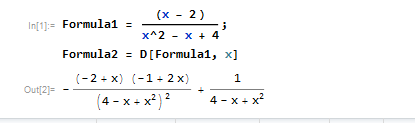## Plotting and Sketching Data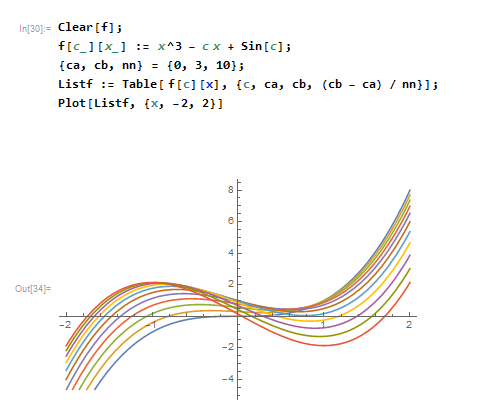## Plotting Derivatives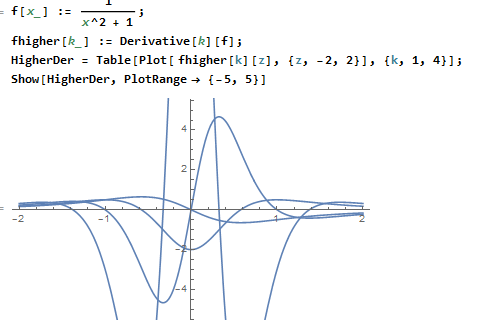## Plotting Points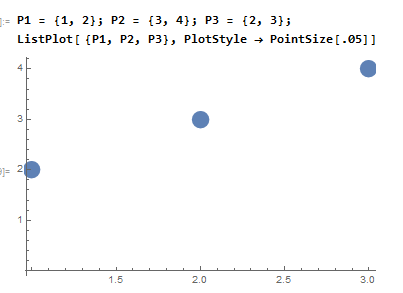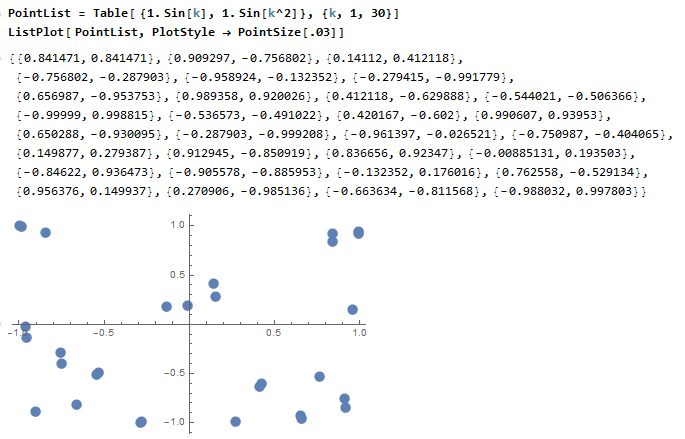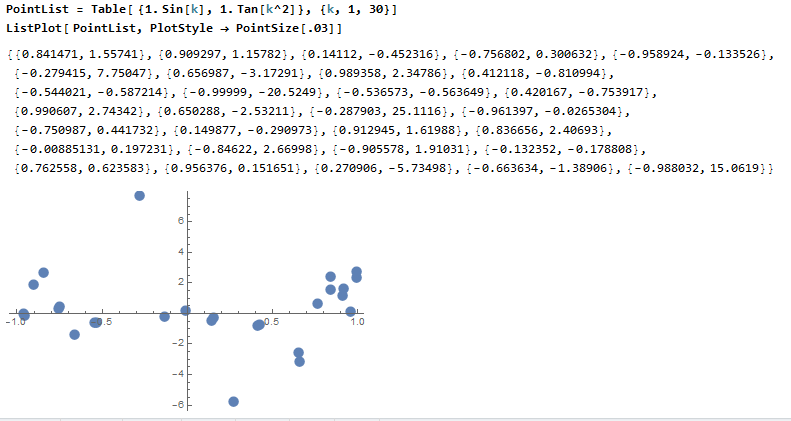## Plotting Animations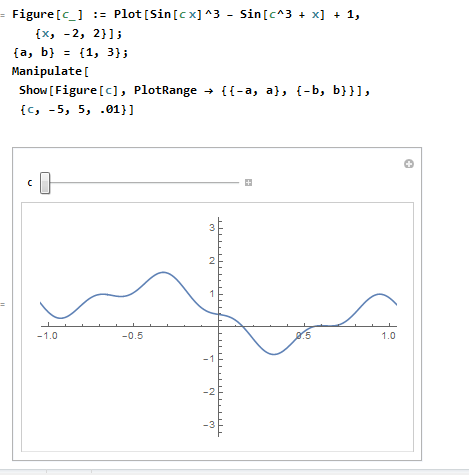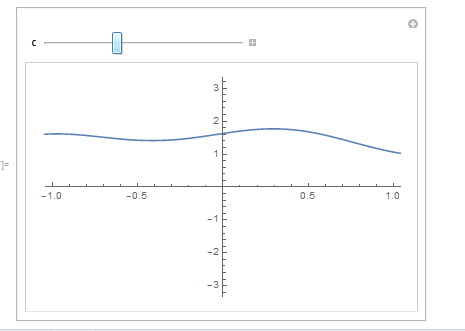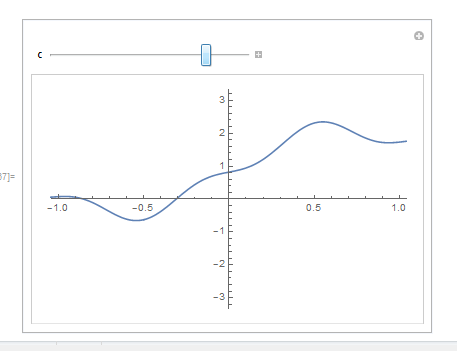## Counter Plots in Mathematica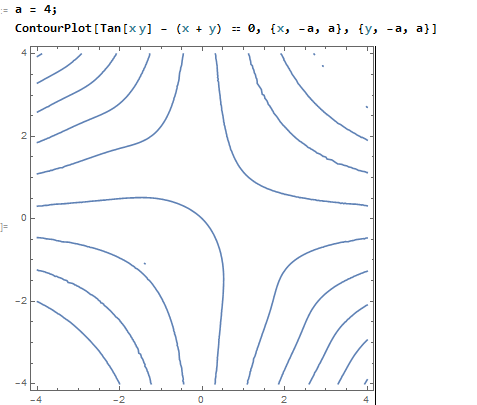## Cartesian Proofs and Plotting in Mathematica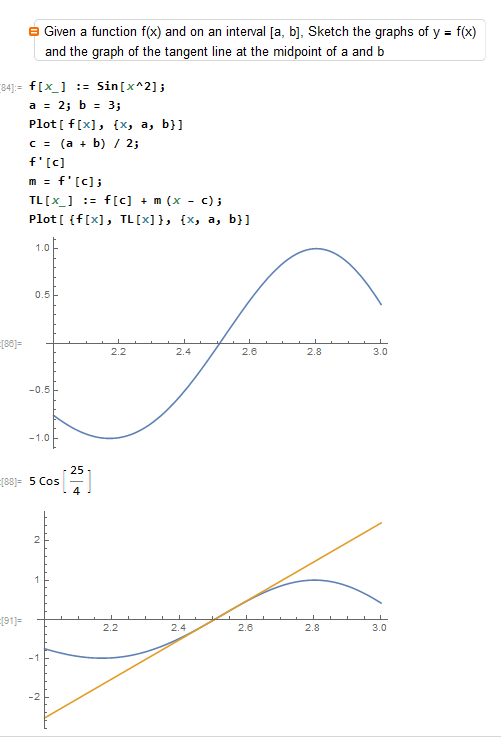## Moved on to Interactive Tutorial for Programmers

• See the lessons here

## Graphics…drawing a Sphere

• Once clicked, it activates a rotation button, so you can rotate it in 3D space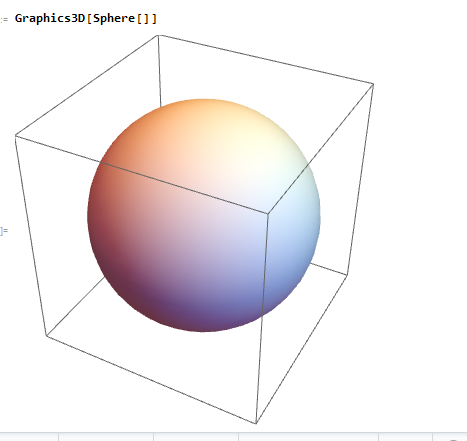## Edge Detection of Sphere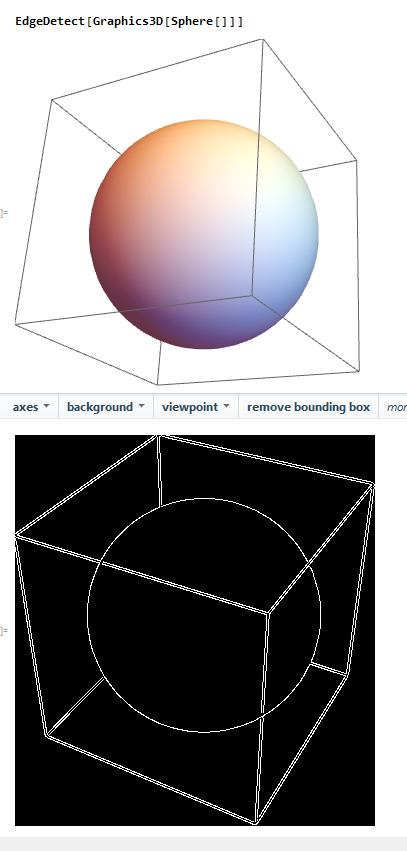## Pattern Matching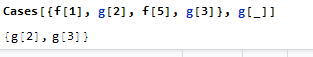## Goals

• Finish up Nanodegree chapter by 9/22 (can do in evenings/ over weekend)
• Finish up Mathematica Interactive tutorial (use all 15 days of trial)
• Send in Application (almost there…by tonight/ tomorrow night latest)
• Start on C++ assignment (can do over weekend/ due Sept 26th)

## Katas

• A Stanton measure:
• Find the number of 1s in a series. Take that count and find the count for that number
``````function stantonMeasure(arr){
var count1 = 0
var total = 0
for (var i = 0; i < arr.length; i++){
if (arr[i] == 1){
count1 = count1 + 1
}
}
for (var i = 0; i < arr.length; i++){
if (arr[i] == count1){
total = total + 1
}
}
}
``````
• Concatenated sum. See if the abs of a number is the same of each digit repeated n times. eg. 198 with n = 2 is 11 + 99 + 88 = 198, which is a Concatenated Sum. Return true if this is true for number given, else false.
``````function checkConcatenatedSum(number, n){
var arr = []
var num = Math.abs(number)
var num1 = num.toString()
var num2 = (num1.split(""))
var total = 0

for (var i = 0; i < num2.length; i++){
arr.push(num2[i].repeat(n))
}
//console.log(arr)
for (var j = 0; j < arr.length; j++){
total = total + parseInt(arr[j])
}
//console.log(total)

if (total == num){
return true
}
else{
return false
}
}
``````
• Given an array, find the max and min and the difference between them. Push to array in order `[min, max, difference]`
``````function differenceInAges(ages){
var arr = []
var ages1 = ages.sort((a, b) => a - b);
var minim = ages1
var maxim = ages1[ages1.length - 1]
var diff = maxim - minim

arr.push(minim)
arr.push(maxim)
arr.push(diff)

return arr
}
``````
• sum of cubes. Given a number, find the total of all the cubes of each number from 0 to n eg. 2 = 1 + 8 = 9
``````def sum_cubes(n):
cube = 0

for i in range(1, n+1):
cube = cube + (i * i * i)

return cube
``````
• Find all occurrences of a number in an array. Return result in array. (use an enum!)
``````def find_all(array, n):
b = [i for i,x in enumerate(array) if x == n]
return b
``````

## …and..that’s it.

Written on August 28, 2017